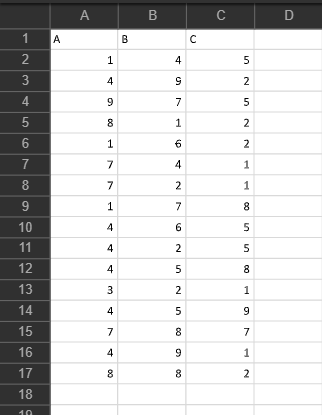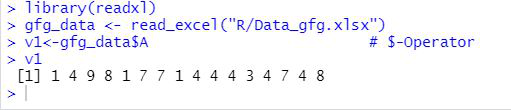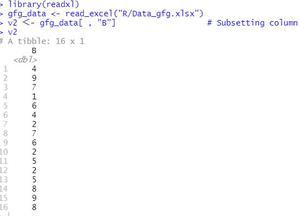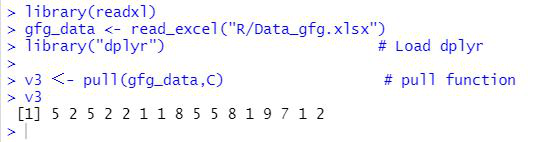Convert an Excel column into a list of vectors in R

• Last Updated : 17 Apr, 2021

In this article, we will be discussing the different approaches to convert the Excel columns to vector in the R Programming language.

File in use:Method 1: Using \$-Operator with the column name

In this method, we will be simply using the \$-operator with the column name and the name of the data read from the excel file. Here, the name of the column name which has to be converted in the vector would be written at the end of the \$-operator and the name of the data read from the excel file will be written before the \$-operator.

Syntax:

dataframe\$column

Example:

R

Output:Method 2: Using the method of Subsetting column

Under this approach user just needs to enter the column name inside the brackets columns with the data. To convert that excel column into vector form. This will only be converting the column to the vector which the user has passed with the brackets along with its data.

Syntax:

dataframe[row_name,column_name]

Example:

R

Output:Method 3: Usingpull function from dplyr library from the R language

Here, the user has to call the pull() function from the dplyr library of the R language and pass the column name which is needed to be converted into the vector form and the name of the datafile read variable.

pull function: This function pull selects a column in a data frame and transforms it into a vector.

Syntax: pull(.data, j)

Parameters:

• .data:-name of the data
• j:-The column to be extracted.

Returns: A vector of the provided column.

Example:

R